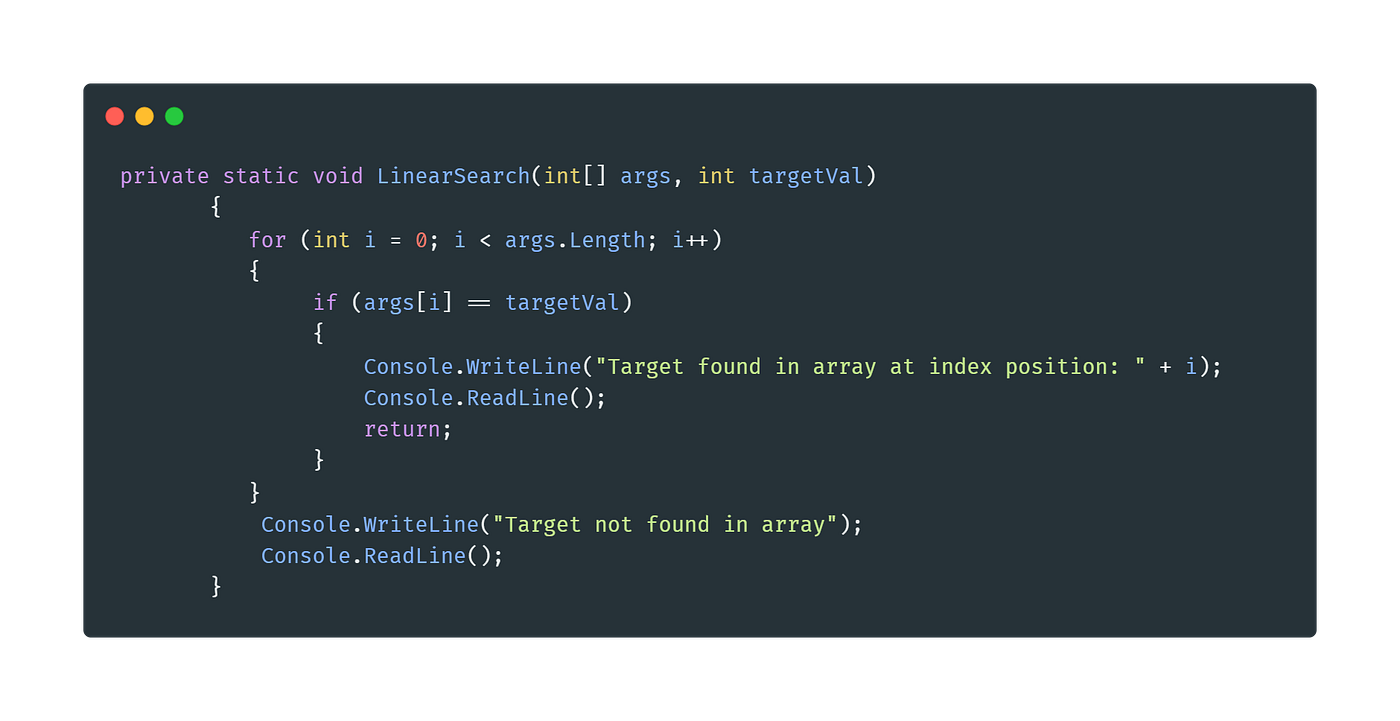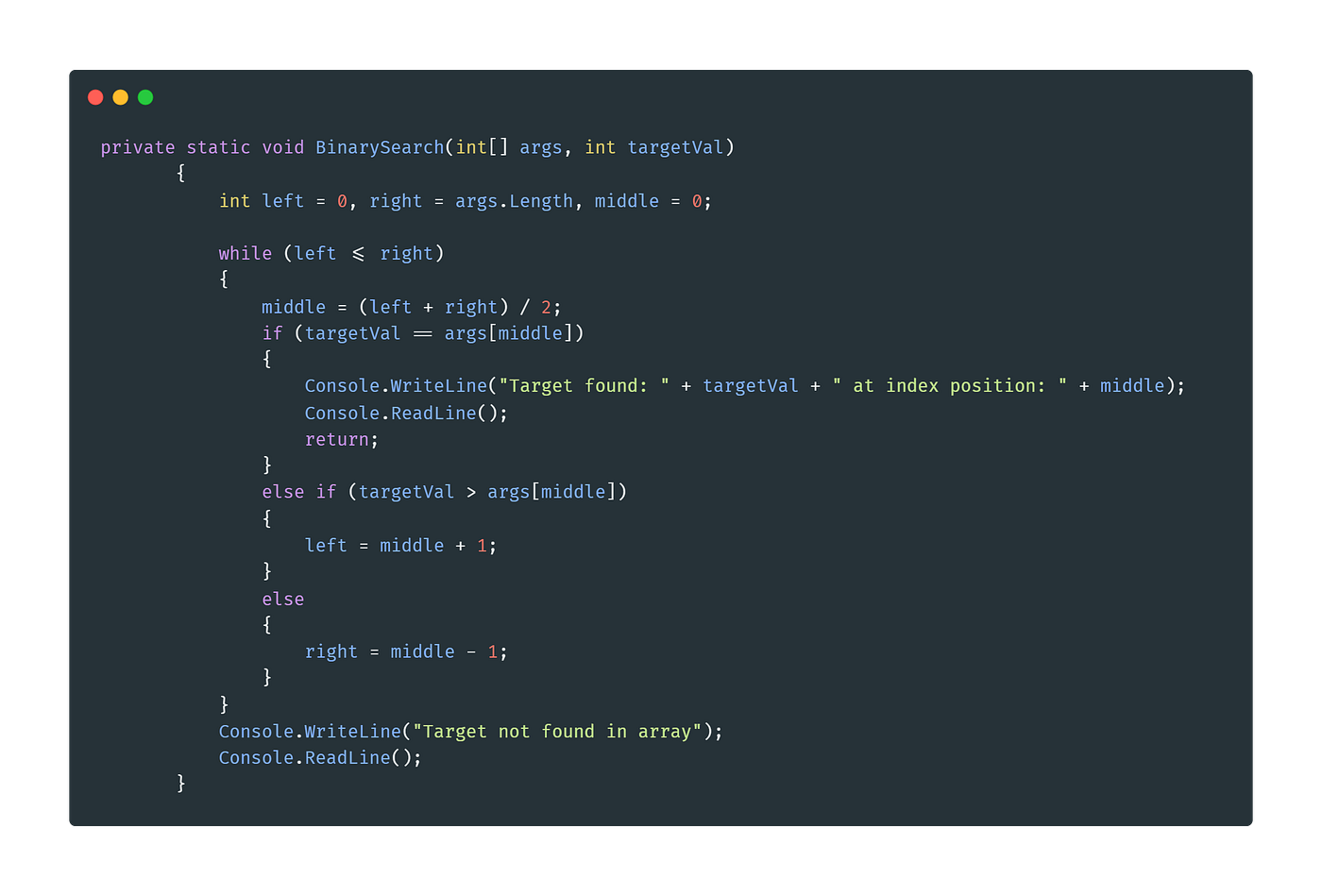# A Brief Introduction to Binary and Linear Searches (C#)

I recently learnt about (and had a little go at using) binary searches in C#, and will do my best to communicate what I’ve learnt so far 😛

Essentially, a binary search is a means of searching an ordered list or array by repeatedly dividing the searchable area in half until we’ve narrowed down and identified the location of a target value. The main reason this is considered so efficient is that we’re not checking every single element in the container (unlike a more linear search), which is particularly important when our inputs are quite large. First, let’s take a look at a linear search:Example of a linear search in a C# program

Here, we’re using a for loop to check each element in an array of integers, starting from the first and working our way up. If/once we find the target value we’re printing a message, letting the user read it, then breaking out of the method. This is quite a common way of looking through a list, but as mentioned this’ll become pretty inefficient when dealing with large arrays, especially when we consider the worst case scenario as we do in “Big O notation”. While the best case would be O(1) (i.e. the target happens to be the first element), the worst case would be O(N) (where N = the length of the array, i.e. the very last element). If it’s the last element of 10 numbers, that’s pretty manageable, but if it’s the last element of, say, 50,000 numbers that’s pretty inefficient. We just had to individually check 50,000 numbers just to find the one we were looking for.

Now let’s take a look at how a binary search compares:Example of a binary search in a C# program

Essentially, what’s happening here is, with each iteration of the while loop, we’re narrowing down the searchable area the target value will be in. To do this, we adjust our “left” and “right” integer variables depending on whether the target value is greater or less than the “args[middle]” value (where our middle value is equal to our left value plus our right one divided by two, which gives us a half-way point rounded down to the closest integer). If our left value is less than our right value, we know the algorithm was unable to find the target value in the array and so the while loop comes to a halt. If we happen to find out target value (where targetVal == args[middle]), then we can use the return keyword to break out of the BinarySearch method.

As for what makes this quite so efficient, imagine if we had that input array of 50,000 elements. In the very first move, the algorithm cuts 25,000 out of the way, then 12,500, then 6,250, and so forth. With some pretty shocking (and ruthless) efficiency this method cuts down the time taken to search through an array drastically.

I’m still getting used to Big O notation, but I believe binary searches have a worst-case performance of O(log N) (which makes them pretty efficient). I’ll briefly cover what a 2D binary search looks like in a subsequent article 😉

--

--